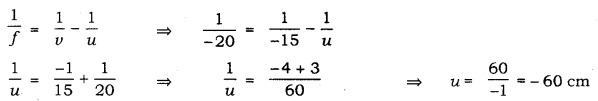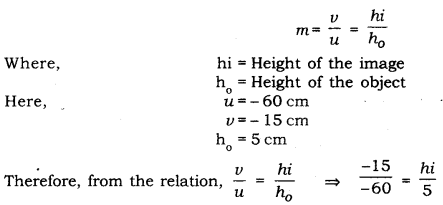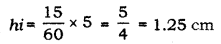# A concave lens has focal length of 20 cm

A concave lens has focal length of 20 cm. At what distance from the lens can a 5 cm tall object be placed so that it forms an image at 15cm from the lens? Also calculate the size of the image formed.

We have,
Image distance (y) = - 15 cm
Focal length (f) = - 20 cm
Now,Therefore, to from an image at 15 cm from the concave lens,the object has placed at a distance 60 cm in front of the lens.
The magnification(m) of the image of an object is related to object distance (u) and image distance (v) asTherefore, the size of the image formed is 1.25 cm.